Home » 4 Types of Distance Metrics in Machine Learning

# 4 Types of Distance Metrics in Machine Learning

Distance metrics are a key part of several machine learning algorithms. These distance metrics are used in both supervised and unsupervised learning, generally to calculate the similarity between data points.

An effective distance metric improves the performance of our machine learning model, whether that’s for classification tasks or clustering.Let’s say we want to create clusters using the K-Means Clustering or k-Nearest Neighbour algorithm to solve a classification or regression problem. How will you define the similarity between different observations here? How can we say that two points are similar to each other?

This will happen if their features are similar, right? When we plot these points, they will be closer to each other in distance.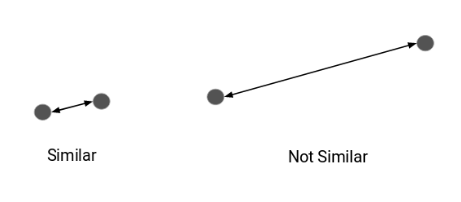Hence, we can calculate the distance between points and then define the similarity between them. Here’s the million-dollar question – how do we calculate this distance and what are the different distance metrics in machine learning?

That’s what we aim to answer in this article. We will walk through 4 types of distance metrics in machine learning and understand how they work in Python.

## 4 Types of Distance Metrics in Machine Learning

1. Euclidean Distance
2. Manhattan Distance
3. Minkowski Distance
4. Hamming Distance

Let’s start with the most commonly used distance metric – Euclidean Distance.

## 1. Euclidean Distance

Euclidean Distance represents the shortest distance between two points.

Most machine learning algorithms including K-Means use this distance metric to measure the similarity between observations. Let’s say we have two points as shown below:

So, the Euclidean Distance between these two points A and B will be: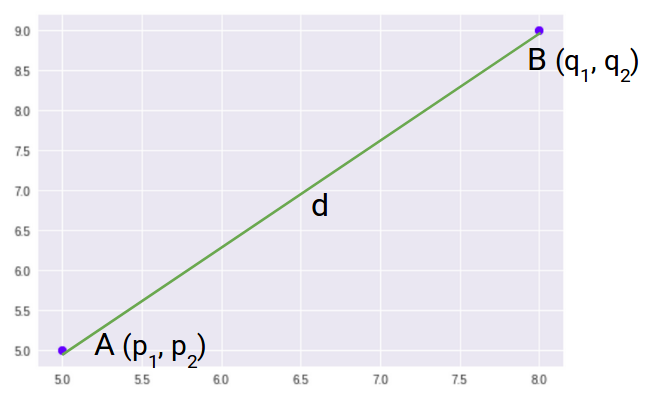Here’s the formula for Euclidean Distance: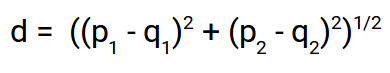We use this formula when we are dealing with 2 dimensions. We can generalize this for an n-dimensional space as: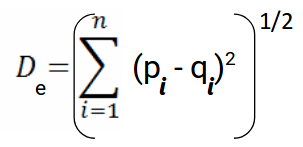Where,

• n = number of dimensions
• pi, qi = data points

Let’s code Euclidean Distance in Python. This will give you a better understanding of how this distance metric works.

We will first import the required libraries. I will be using the SciPy library that contains pre-written codes for most of the distance functions used in Python: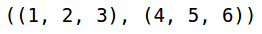These are the two sample points which we will be using to calculate the different distance functions. Let’s now calculate the Euclidean Distance between these two points: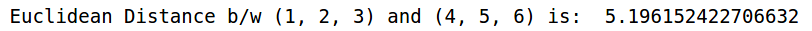This is how we can calculate the Euclidean Distance between two points in Python. Let’s now understand the second distance metric, Manhattan Distance.

## 2. Manhattan Distance

Manhattan Distance is the sum of absolute differences between points across all the dimensions.

We can represent Manhattan Distance as:

Since the above representation is 2 dimensional, to calculate Manhattan Distance, we will take the sum of absolute distances in both the x and y directions. So, the Manhattan distance in a 2-dimensional space is given as:

And the generalized formula for an n-dimensional space is given as:

Where,

• n = number of dimensions
• pi, qi = data points

Now, we will calculate the Manhattan Distance between the two points: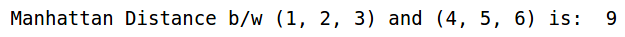Note that Manhattan Distance is also known as city block distance. SciPy has a function called cityblock that returns the Manhattan Distance between two points.

Let’s now look at the next distance metric – Minkowski Distance.

## 3. Minkowski Distance

Minkowski Distance is the generalized form of Euclidean and Manhattan Distance.

The formula for Minkowski Distance is given as:

Here, p represents the order of the norm. Let’s calculate the Minkowski Distance of the order 3: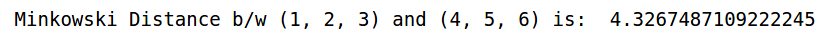The p parameter of the Minkowski Distance metric of SciPy represents the order of the norm. When the order(p) is 1, it will represent Manhattan Distance and when the order in the above formula is 2, it will represent Euclidean Distance.

Let’s verify that in Python: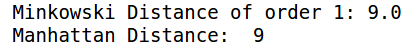Here, you can see that when the order is 1, both Minkowski and Manhattan Distance are the same. Let’s verify the Euclidean Distance as well: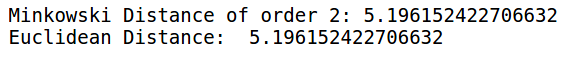When the order is 2, we can see that Minkowski and Euclidean distances are the same.

So far, we have covered the distance metrics that are used when we are dealing with continuous or numerical variables. But what if we have categorical variables? How can we decide the similarity between categorical variables? This is where we can make use of another distance metric called Hamming Distance.

## 4. Hamming Distance

Hamming Distance measures the similarity between two strings of the same length. The Hamming Distance between two strings of the same length is the number of positions at which the corresponding characters are different.

Let’s understand the concept using an example. Let’s say we have two strings:

“euclidean” and “manhattan”

Since the length of these strings is equal, we can calculate the Hamming Distance. We will go character by character and match the strings. The first character of both the strings (e and m respectively) is different. Similarly, the second character of both the strings (u and a) is different. and so on.

Look carefully – seven characters are different whereas two characters (the last two characters) are similar:

Hence, the Hamming Distance here will be 7. Note that larger the Hamming Distance between two strings, more dissimilar will be those strings (and vice versa).

Let’s see how we can compute the Hamming Distance of two strings in Python. First, we’ll define two strings that we will be using:

These are the two strings “euclidean” and “manhattan” which we have seen in the example as well. Let’s now calculate the Hamming distance between these two strings: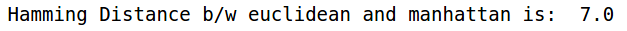As we saw in the example above, the Hamming Distance between “euclidean” and “manhattan” is 7. We also saw that Hamming Distance only works when we have strings of the same length.

Let’s see what happens when we have strings of different lengths: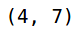You can see that the lengths of both the strings are different. Let’s see what will happen when we try to calculate the Hamming Distance between these two strings: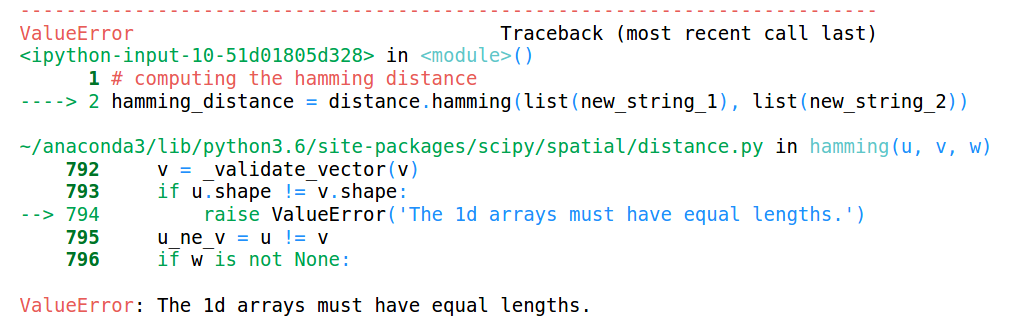This throws an error saying that the lengths of the arrays must be the same. Hence, Hamming distance only works when we have strings or arrays of the same length.

These are some of the similarity measures or the distance matrices that are generally used in Machine Learning.

You can also read this article on our Mobile APP